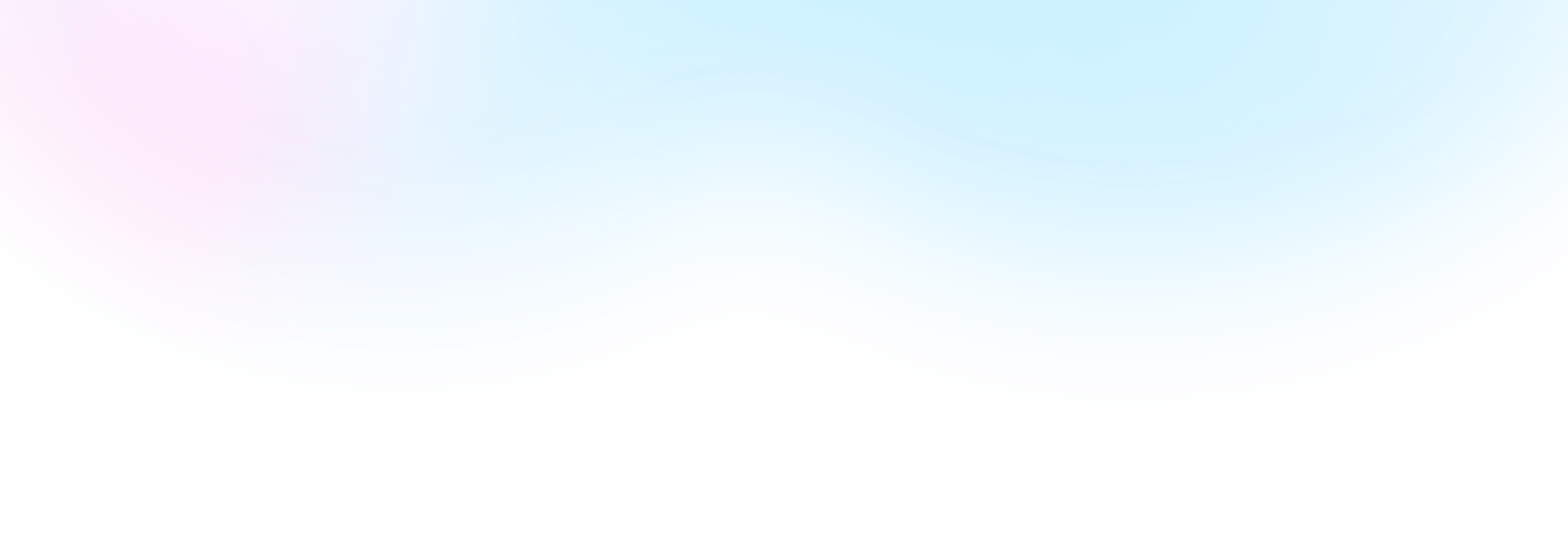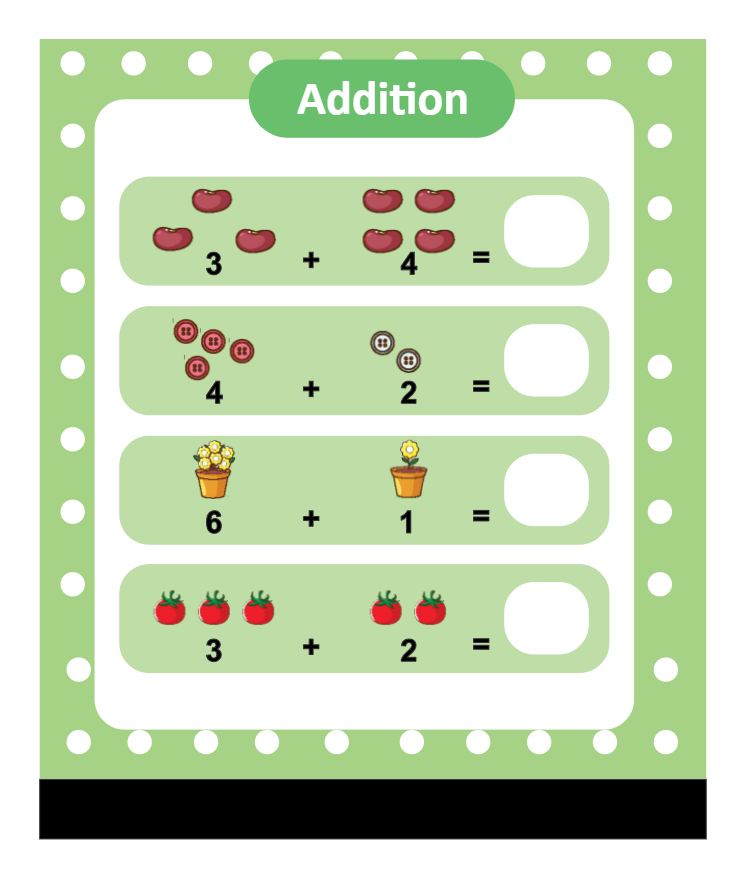Template Community / Math Worksheet Addition TemplateKiraaaa
Published on 2022-02-09
Edit OnlineIn mathematics, addition is adding two more items together. This free addition worksheet is great for students who are learning basic math. In the math worksheets addition template, we see how students learn basic mathematics by looking at cool and funny pictures. The mat will be explained in the easy language in the worksheets so the younger children can easily understand it. As illustrated from the math worksheets addition, an additional sentence is a mathematical expression that shows two or more values together. You can use EdrawMax to create such math-related addition problems for your preschool and kindergarten students. With EdrawMax, you can also share the worksheets with the template community.
Tag
science diagram
Mathematics Diagram
Report
0
27PostRecommended Templates